### Properties of Common Gases/Steam and Moist Air with Temperature

The symbol for the Universal Gas Constant is Ru= 8.314 J/mol.K  (0.0831 bar dm3 mol-1 K-1).  The Specific-Heat Capacity, C, is defined as the amount of heat required to raise the temperature by 1K per mole or per kg. The Specific Heat Capacity is measured and reported at constant pressure (Cp) or constant volume (Cv) conditions.   For monoatomic gases Cp=2.5Ru J/mol.K  and Cv=1.5Ru J/mol.K, respectively. The ratio of the two specific heats is called the adiabatic ratio of the gas.  The symbol for the adiabatic ratio is g (gamma) and is equal to 1.667 for all monoatomic ideal gases like He, Ar, and Ne.   Unless exceeding 10 Bar, the use pressure of many commonly used process gasses can be treated as being ideal which means that individual molecules and atoms do not feel any significant bond energy from other molecules or atoms in the same gas. The specific heat changes as shown below with the number of atoms in a molecule of a gas.  For diatomic gases, the Cp, Cv, and g are 3.5Ru, 2.5Ru, and 1.4 respectively.  Note how the distribution of energy to the various rotational modes for diatomic gases over monoatomic gases has added 0.5Ru per mode, to the gas-specific-heat capacity.  This is a consequence of the equipartition theorem.  So far we have only assumed that the gasses are at a low temperature where the vibrational mode has not yet been accessed.  A vibrational mode contributes twice as much to the heat capacity as a translational mode, but only if it is accessible.  When vibrational modes are included e.g. in a solid the calculation of specific is not simple. In a solid, the frequencies of the vibration (vibrionic modes) span a range. At lower temperatures, only the low-frequency modes are available to take up heat. For condensed matter, the experimental heat capacity is noted to approach a  limit of 3Ru= 24.9 J/mol.K  for simple substances like simple metallic materials.  For molecular or ordered solids it will be more e.g ~ 6Ru= 49.884 J/mol.K for dimolecular solids.  When this happens one may infer that all of the vibrational mode possibilities are active (for taking up the energy).   For solids and liquids the two specific heats (Cp and Cv) are almost equal  (aluminum is 24.2 J/mol.K, titanium is 26 J/mol.K,  Iron is 25.1 J/mol.K).  For ideal gases, the specific heat of the gas is independent of temperature.   Note that many of the specific heat values measured or calculated for polyatomic atomic configurations are temperature dependent. The evidence regarding the behavior of vibrational energy in periodic (orderly) solids is that the collective vibrational modes can accept energy only in discrete amounts, and these quanta of energy are labeled phonons (lattice vibration states). Like the photons of electromagnetic energy, the phonons obey Bose-Einstein statistics.  At very low temperatures only the specific contributions from electrons are important and so the Fermi Dirac statistics apply.  As an approximation, the dependence of the specific heat with temperature in Kelvin is T^3 (for the condensed matter) at very low temperatures.  The specific heat dependence is linear at higher temperatures before reaching a limit when all the vibrational states are fully active (and there is no further dependence on temperature).  If a phase change should occur, the specific heat is not defined at the phase change temperature.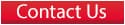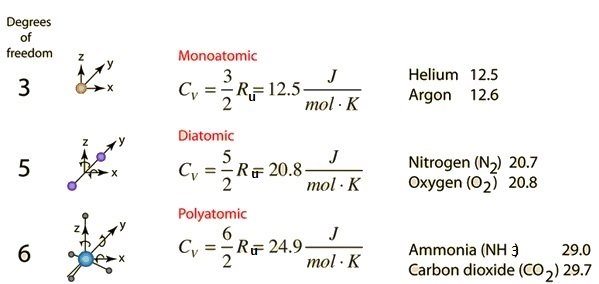Cp= Cv+Ru. Ru is  8.314 J/mol.K (universal constant) for all substances when expressed in the units J/mol.K.  The material-specific gas constant R, however when expressed in the units of kJ/kg.K is dependent on the materials.  R is equal to Ru/M where M is the molecular weight in moles/kg.

R, the gas constant expressed in units of kJ/kg.K for common gases are given below.

Air                   0.2870  kJ/kg.K

Hydrogen         4.1240  kJ/kg.K

Argon              0.2081  kJ/kg.K

Nitrogen          0.2986  kJ/kg.K

Oxygen            0.2598 kJ/kg.K

Steam              0.4615   kJ/kg.K

Most process gases can be considered close to ideal at high temperatures and one atmospheric pressure.     In the units, kJ/kg.K the gas-specific heat capacity @ 300K is given below.  If the gas is truly ideal, the Specific Heat Capacity is temperature independent. Note hydrogen behaves more like a monoatomic gas at low temperatures.

Air                   Cp= 1.005  kJ/kg.K      Cv=0.718 kJ/kg.K     Density @ STP 1.29kg/m3

Hydrogen         Cp=14.307   kJ/kg.K    Cv=10.183  kJ/kg.K  Density @ STP 0.09kg/m3

Argon              Cp=0.5203  kJ/kg.K    Cv=0.3122 kJ/kg.K    Density @ STP 1.78kg/m3

CO2                Cp=0.8443  kJ/kg.K    Cv=0.6553 kJ/kg.K    Density @ STP 1.96kg/m3

Nitrogen           Cp=1.0396  kJ/kg.K    Cv=0.743 kJ/kg.K     Density @ STP 1.25kg/m3

Oxygen             Cp=0.918  kJ/kg.K     Cv=0.658 kJ/kg.K     Density @ STP 1.43kg/m3

Steam               Cp=1.8723  kJ/kg.K    Cv=1.4108 kJ/kg.K    – see steam tables.

At IUPAC standard temperature and pressure (0 °C and 101.325 kPa), dry air has a density of 1.2754 kg/m3.  At 20 °C and 101.325 kPa, dry air has a density of 1.2041 kg/m3  i.e.at 70 °F and 14.696 psia, dry air has a density of 0.074887lbm/ft3.

Both energy and enthalpy are measured from a base temperature.  Therefore only a change in properties of this kind is measurable.  The change in enthalpy (H) is DH = Mass. Cp. (Change in temperature in Kelvin).  The Enthalpy per kg (or specific enthalpy) is given the symbol h.  The change in internal energy (U) is D= Mass. Cv. (Change in temperature in Kelvin).  The Internal Energy per kg (or specific internal energy) is given the symbol u.

The table below gives Enthalpy per kg (h) and Energy per kg (u), and Density of Air, at various temperatures achieved by the Airtorch™.  Base ~ 0K.

Temperature of Air in Centigrade (°C).  For Density and Enthalpy and Energy use a base of 0K

Change in Enthalpy
– h –
(kJ/kg) IT may be calculated by subtracting between  ROW CHANGE CHANGE

Change in Internal Energy
–
(kJ/kg) IT may be calculated by subtracting between  ROW DENSITY

Density

Kg/m3 at corresponding temperature in  Centigrade (°C) given in THE first column

200

200

143

0.7459

300

300

214

0.6158

400

401

286

0.5243

500

503

359

0.4565

600

607

435

0.4042

700

713

512

0.3627

800

822

592

0.3289

900

933

675

0.3008

1000

1046

759

0.2772

1500

1636

1205

0.1553

Please note that the above approximations should be verified from standard texts before use. Unintended typographical errors are possible.

Click for Steam Calculator

What is humidity?

Other Important Fluid Properties

Other important properties of a gas are the dynamic-viscosity (defined below), kinematic-viscosity, and Prandtl number (defined below).   For an ideal gas, these are independent of temperature.  The Prandtl Number is often used in heat transfer and free and forced convection calculations. It depends on the fluid properties.  The Pr ranges from 0.7 – 1.0 for common process gasses, and Pr ranges from 0.001 – 0.03 for simple liquid metals.   For polar liquids like water and oils, the Pr ranges from 1 – 2200  (the lower-end being for water and the higher-end for high molecular weight viscous oils).   The Prandtl Number is a dimensionless number that is the ratio of momentum diffusivity (kinematic viscosity) and thermal diffusivity.  This number may as you imagine give a sense of comparison of viscous and thermal forces required for an objective, as well as indicate what carries shear force to what carrier the thermal (heat) transport in a molecular sense!

Pr = v / α  or  Pr = μ cp / k  where;

cp= specific heat capacity (J/kg K) at constant pressure,

k = thermal conductivity (W/m K)

μ = absolute or dynamic viscosity (kg/ms).  The dynamic ( or absolute) viscosity is the tangential force per unit area required to move one horizontal plane of a fluid concerning the other at unit velocity when maintained a unit distance apart.

α = thermal diffusivity (m2/s)

v = kinematic viscosity or momentum diffusivity (m2/s).  Kinematic Viscosity is the ratio of absolute or dynamic viscosity to density.  Kinematic viscosity can be obtained by dividing the absolute viscosity of a fluid by density.

Pr = Prandtl’s number. (Note dimensionless).

Viscosity of Steam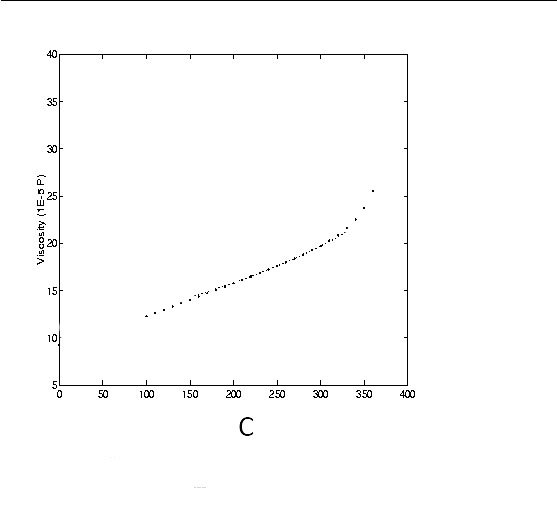Flow Rate of 0.3g/min (~0.3ml/min) Water Conversion at Various Temperatures Constant Pressure at 1 bar with Water Inlet g/min= 0.3 Temp (°C) Specific Volume v (m3/kg) Volume flow rate(mL/min) (steam) 100 1.6959 509 150 1.9367 581 200 2.1724 652 250 2.4062 722 300 2.6389 792 400 3.1027 931 500 3.5655 1070 600 4.0279 1208 700 4.4900 1347 800 4.9519 1486 900 5.4137 1624 1000 5.8755 1763 1100 6.3372 1901 1200 6.7988 2040 1300 7.2605 2178

Equations for the physical properties of moist air

Water vapor pressure

In a closed container partly filled with water, there will be some water vapor in the space above the water. The concentration of water vapor depends only on the temperature. It is not dependent on the amount of water and is only very slightly influenced by the pressure of air in the container.  The water vapor exerts pressure on the walls of the container. The empirical equations given below give a good approximation to the saturation water vapor pressure at temperatures within the limits of the earth’s climate.

Saturation vapor pressure, ps, in pascals:
ps = 610.78 *exp( t / ( t + 238.3 ) *17.2694 )
where is the temperature in degrees Celsius

The svp below freezing can be corrected after using the equation above, thus:
ps ice = -4.86 + 0.855*ps + 0.000244*ps2

The next formula gives a direct result for the saturation vapor pressure over ice:
ps ice = exp( -6140.4 / ( 273 + t ) + 28.916 )

The pascal is the SI unit of pressure = newtons / m2. Atmospheric pressure is about 100,000 Pa (standard atmospheric pressure is defined as 101,300 Pa).

Water vapor concentration

The relationship between vapor pressure and concentration is defined for any gas by the equation:
p = nRT/V
p is the pressure in Pa, V is the volume in cubic meters, T is the temperature in degrees Kelvin (degrees Celsius + 273.16), n is the quantity of gas expressed in molar mass ( 0.018 kg in the case of water ), R is the gas constant: 8.31 Joules/mol.K

To convert the water vapor pressure to concentration in kg/m3: ( Kg / 0.018 ) / V = p / RT

kg/m3 = 0.002166 *p / ( t + 273.16 )   where p is the actual vapor pressure

Relative Humidity

The Relative Humidity (RH) is the ratio of the actual water vapor pressure to the saturation water vapor pressure at the prevailing temperature.

RH = p/ps

RH is usually expressed as a percentage rather than as a fraction.

The RH is a ratio. It does not define the water content of the air unless the temperature is given. The reason RH is so much used in conservation is that most organic materials have an equilibrium water content that is mainly determined by the RH and is only slightly influenced by temperature.

Notice that air is not involved in the definition of RH. Airless space can have an RH. Air is the transporter of water vapor in the atmosphere and air conditioning systems, so the phrase “RH of the air” is commonly used, and only occasionally misleading. The independence of RH from atmospheric pressure is not important on the ground, but it does have some relevance to calculations concerning air transport of works of art and conservation by freeze-drying.

The Dew Point

The water vapor content of air is often quoted as a dew point. This is the temperature to which the air must be cooled before dew condenses from it. At this temperature, the actual water vapor content of the air is equal to the saturation water vapor pressure. The dew point is usually calculated from the RH. The first one calculates ps, the saturation vapor pressure at the ambient temperature. The actual water vapor pressure, pa, is:

pa= ps * RH% / 100

The next step is to calculate the temperature at which pa would be the saturation vapor pressure. This means running backward the equation given above for deriving saturation vapor pressure from temperature:

Let w = ln ( pa/ 610.78 )
Dew point = w *238.3 / ( 17.294 – w )

This calculation is often used to judge the probability of condensation on windows and within walls and roofs of humidified buildings.

The dew point can also be measured directly by cooling a mirror until it fogs. The RH is then given by the ratio

RH = 100 * ps dewpoint/psambient

The concentration of water vapor in the air

It is sometimes convenient to quote water vapor concentration as kg/kg of dry air. This is used in air conditioning calculations and is quoted on psychrometric charts. The following calculations for water vapor concentration in air apply at ground level.

Dry air has a molar mass of 0.029 kg. It is denser than water vapor, which has a molar mass of 0.018 kg. Therefore, humid air is lighter than dry air. If the total atmospheric pressure is P and the water vapor pressure is p, the partial pressure of the dry air component is P – p. The weight ratio of the two components, water vapor, and dry air is:

kg water vapor / kg dry air = 0.018 *p / ( 0.029 *(P – p ) )
= 0.62 *p / (P – p )

At room temperature P – p is nearly equal to P, which at ground level is close to 100,000 Pa, so, approximately:

kg water vapor / kg dry air = 0.62 *10-5 *p

Thermal properties of damp air

The heat content, usually called the enthalpy, of air, rises with increasing water content. This hidden heat, called latent heat by air conditioning engineers, has to be supplied or removed to change the relative humidity of the air, even at a constant temperature. This is relevant to conservators. The transfer of heat from an air stream to a wet surface, which releases water vapor to the airstream at the same time as it cools it, is the basis for psychrometry and many other microclimatic phenomena. Control of heat transfer can be used to control the drying and wetting of materials during conservation treatment.

The enthalpy of dry air is not known. Air at zero degrees Celsius is defined to have zero enthalpies. The enthalpy, in kJ/kg, at any temperature, t, between 0 and 60C is approximate:

h = 1.007t – 0.026   below zero: h = 1.005t

The enthalpy of liquid water is also sometimes defined to be zero at zero degrees Celsius. To turn liquid water to vapor at the same temperature requires a very considerable amount of heat energy: 2501 kJ/kg at 0C.

At temperature t the heat content of water vapor is:

hw = 2501 + 1.84

Notice that water vapor, once generated, also requires more heat than dry air to raise its temperature further: 1.84 kJ/kg.C against about 1 kJ/kg.C for dry air.

The enthalpy of moist air, in kJ/kg, is therefore:

h = (1.007*t – 0.026) + g*(2501 + 1.84*t)
g is the water content (specific humidity) in kg/kg of dry air

The Psychrometer

The final formula in this collection is the psychrometric equation. The psychrometer is the nearest to an absolute method of measuring RH that the conservator ever needs. It is more reliable than electronic devices because it depends on the calibration of thermometers or temperature sensors, which are much more reliable than electrical RH sensors. The only limitation to the psychrometer is that it is difficult to use in confined spaces (not because it needs to be whirled around but because it releases water vapor).

The psychrometer, or wet and dry bulb thermometer, responds to the RH of the air in this way:

Unsaturated air evaporates water from the wet wick. The heat required to evaporate the water into the air stream is taken from the air stream, which cools in contact with the wet surface, thus cooling the thermometer beneath it. An equilibrium wet surface temperature is reached which is very roughly halfway between ambient temperature and dew point temperature.

The air’s potential to absorb water is proportional to the difference between the mole fraction, ma, of water vapor in the ambient air and the mole fraction, mw, of water vapor in the saturated air at the wet surface. It is this capacity to carry away water vapor that drives the temperature down to, tw, the wet thermometer temperature, from the ambient temperature ta :
(mw – ma) = B(ta- tw)
B is a constant, whose numerical value can be derived theoretically by some rather complicated physics (see the reference below).

The water vapor concentration is expressed here as a mole fraction in air, rather than as vapor pressure. Air is involved in the psychrometric equation because it brings the heat required to evaporate water from the wet surface. The constant B is therefore dependent on total air pressure, P. However the mole fraction, m, is simply the ratio of vapor pressure p to total pressure P:  p/P. The air pressure is the same for both ambient air and air in contact with the wet surface, so the constant B can be modified to a new value, A, which incorporates the pressure, allowing the molar fractions to be replaced by the corresponding vapor pressures:

pw – pa= A* ( ta- tw)

The relative humidity (as already defined) is the ratio of pa, the actual water vapor pressure of the air, tops, and the saturation water vapor pressure at ambient temperature.

RH% = 100 *pa/ ps = 100 *( pw – ( ta- tw) * 63) / ps
When the wet thermometer is frozen the constant changes to 56

The psychrometric constant is taken from: R.G.Wylie & T. Lalas, “Accurate psychrometer coefficients for wet and ice-covered cylinders in laminar transverse air streams”, in Moisture and Humidity 1985, published by the Instrument Society of America, pp 37 – 56. These values are slightly lower than those in general use. There are tables and slide rules for calculating RH from the psychrometer but a programmable calculator is very handy for this job. Alternatively, click on the calculator.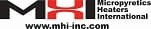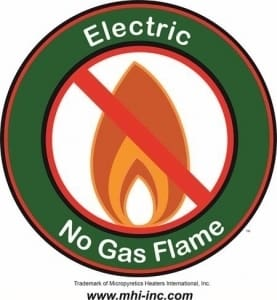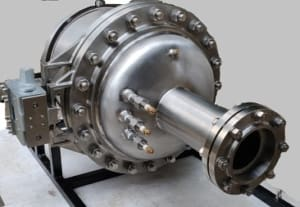SH 1000C